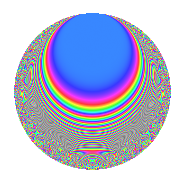# Properties

 Label 862.2.aLevel 862 Weight 2 Character orbit a Rep. character $$\chi_{862}(1,\cdot)$$ Character field $$\Q$$ Dimension 35 Newforms 11 Sturm bound 216 Trace bound 5

# Related objects

## Defining parameters

 Level: $$N$$ = $$862 = 2 \cdot 431$$ Weight: $$k$$ = $$2$$ Character orbit: $$[\chi]$$ = 862.a (trivial) Character field: $$\Q$$ Newforms: $$11$$ Sturm bound: $$216$$ Trace bound: $$5$$ Distinguishing $$T_p$$: $$3$$, $$5$$

## Dimensions

The following table gives the dimensions of various subspaces of $$M_{2}(\Gamma_0(862))$$.

Total New Old
Modular forms 110 35 75
Cusp forms 107 35 72
Eisenstein series 3 0 3

The following table gives the dimensions of the cuspidal new subspaces with specified eigenvalues for the Atkin-Lehner operators and the Fricke involution.

$$2$$$$431$$FrickeDim.
$$+$$$$+$$$$+$$$$8$$
$$+$$$$-$$$$-$$$$10$$
$$-$$$$+$$$$-$$$$9$$
$$-$$$$-$$$$+$$$$8$$
Plus space$$+$$$$16$$
Minus space$$-$$$$19$$

## Trace form

 $$35q - q^{2} - 4q^{3} + 35q^{4} - 6q^{5} - 4q^{6} - 8q^{7} - q^{8} + 31q^{9} + O(q^{10})$$ $$35q - q^{2} - 4q^{3} + 35q^{4} - 6q^{5} - 4q^{6} - 8q^{7} - q^{8} + 31q^{9} - 6q^{10} - 4q^{12} + 35q^{16} - 2q^{17} + 3q^{18} - 8q^{19} - 6q^{20} + 4q^{23} - 4q^{24} + 21q^{25} - 4q^{27} - 8q^{28} - 2q^{29} + 4q^{30} - 28q^{31} - q^{32} + 12q^{33} - 14q^{34} - 4q^{35} + 31q^{36} - 24q^{37} - 4q^{38} + 16q^{39} - 6q^{40} - 6q^{41} - 8q^{42} - 18q^{43} - 42q^{45} - 4q^{46} - 20q^{47} - 4q^{48} + 7q^{49} - 15q^{50} + 28q^{51} + 22q^{53} - 40q^{54} - 12q^{55} - 20q^{57} - 22q^{58} - 18q^{61} - 4q^{62} - 4q^{63} + 35q^{64} - 12q^{65} - 8q^{66} - 14q^{67} - 2q^{68} + 16q^{69} - 28q^{70} - 12q^{71} + 3q^{72} - 42q^{73} + 4q^{74} + 4q^{75} - 8q^{76} - 12q^{77} + 16q^{78} - 4q^{79} - 6q^{80} + 75q^{81} - 26q^{82} + 38q^{83} - 4q^{85} + 2q^{86} + 24q^{87} + 6q^{89} + 14q^{90} - 24q^{91} + 4q^{92} + 36q^{93} - 12q^{94} + 52q^{95} - 4q^{96} - 22q^{97} + 23q^{98} + 12q^{99} + O(q^{100})$$

## Decomposition of $$S_{2}^{\mathrm{new}}(\Gamma_0(862))$$ into irreducible Hecke orbits

Label Dim. $$A$$ Field CM Traces A-L signs $q$-expansion
$$a_2$$ $$a_3$$ $$a_5$$ $$a_7$$ 2 431
862.2.a.a $$1$$ $$6.883$$ $$\Q$$ None $$-1$$ $$-3$$ $$-1$$ $$-2$$ $$+$$ $$+$$ $$q-q^{2}-3q^{3}+q^{4}-q^{5}+3q^{6}-2q^{7}+\cdots$$
862.2.a.b $$1$$ $$6.883$$ $$\Q$$ None $$-1$$ $$1$$ $$-1$$ $$-2$$ $$+$$ $$+$$ $$q-q^{2}+q^{3}+q^{4}-q^{5}-q^{6}-2q^{7}+\cdots$$
862.2.a.c $$1$$ $$6.883$$ $$\Q$$ None $$1$$ $$-1$$ $$-3$$ $$2$$ $$-$$ $$-$$ $$q+q^{2}-q^{3}+q^{4}-3q^{5}-q^{6}+2q^{7}+\cdots$$
862.2.a.d $$1$$ $$6.883$$ $$\Q$$ None $$1$$ $$-1$$ $$1$$ $$-2$$ $$-$$ $$-$$ $$q+q^{2}-q^{3}+q^{4}+q^{5}-q^{6}-2q^{7}+\cdots$$
862.2.a.e $$1$$ $$6.883$$ $$\Q$$ None $$1$$ $$0$$ $$2$$ $$4$$ $$-$$ $$+$$ $$q+q^{2}+q^{4}+2q^{5}+4q^{7}+q^{8}-3q^{9}+\cdots$$
862.2.a.f $$1$$ $$6.883$$ $$\Q$$ None $$1$$ $$1$$ $$-3$$ $$2$$ $$-$$ $$+$$ $$q+q^{2}+q^{3}+q^{4}-3q^{5}+q^{6}+2q^{7}+\cdots$$
862.2.a.g $$2$$ $$6.883$$ $$\Q(\sqrt{5})$$ None $$2$$ $$0$$ $$2$$ $$2$$ $$-$$ $$+$$ $$q+q^{2}-\beta q^{3}+q^{4}+q^{5}-\beta q^{6}+(1+\cdots)q^{7}+\cdots$$
862.2.a.h $$5$$ $$6.883$$ 5.5.181057.1 None $$5$$ $$5$$ $$3$$ $$-3$$ $$-$$ $$+$$ $$q+q^{2}+(1-\beta _{2})q^{3}+q^{4}+(1+\beta _{3})q^{5}+\cdots$$
862.2.a.i $$6$$ $$6.883$$ 6.6.11017801.1 None $$-6$$ $$-2$$ $$-2$$ $$3$$ $$+$$ $$+$$ $$q-q^{2}-\beta _{1}q^{3}+q^{4}+(-\beta _{2}+\beta _{4}-\beta _{5})q^{5}+\cdots$$
862.2.a.j $$6$$ $$6.883$$ 6.6.9783113.1 None $$6$$ $$-8$$ $$-8$$ $$-9$$ $$-$$ $$-$$ $$q+q^{2}+(-1+\beta _{2}+\beta _{4})q^{3}+q^{4}+(-2+\cdots)q^{5}+\cdots$$
862.2.a.k $$10$$ $$6.883$$ $$\mathbb{Q}[x]/(x^{10} - \cdots)$$ None $$-10$$ $$4$$ $$4$$ $$-3$$ $$+$$ $$-$$ $$q-q^{2}+\beta _{5}q^{3}+q^{4}+(-\beta _{7}-\beta _{9})q^{5}+\cdots$$

## Decomposition of $$S_{2}^{\mathrm{old}}(\Gamma_0(862))$$ into lower level spaces

$$S_{2}^{\mathrm{old}}(\Gamma_0(862)) \cong$$ $$S_{2}^{\mathrm{new}}(\Gamma_0(431))$$$$^{\oplus 2}$$